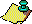Contents Next: Behavior of the Up: The 3x+1 problem. Previous: The 3x+1 problem.

The following heuristic probabilistic argument supports the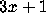Conjecture (see ). Pick an odd integer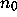at random and iterate the function T until another odd integer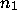occurs. Thenof the time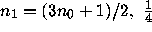of the time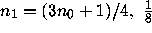of the time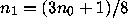, and so on. If one supposes that the function T is sufficiently ``mixing'' that successive odd integers in the trajectory of n behave as though they were drawn at random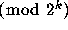from the set of odd integersfor all k, then the expected growth in size between two consecutive odd integers in such a trajectory is the multiplicative factor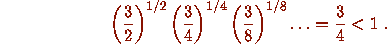Consequently this heuristic argument suggests that on average the iterates in a trajectory tend to shrink in size, so that divergent trajectories should not exist. Furthermore it suggests that the total stopping time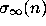is (in some average sense) a constant multiple of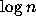. (Click here for more.)

From the viewpoint of this heuristic argument, the central difficulty of theproblem lies in understanding in detail the ``mixing'' properties of iterates of the function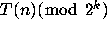for all powers of 2. The function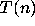does indeed have some ``mixing'' properties given by Theorems B and K below; these are much weaker than what one needs to settle theConjecture.Contents Next: Behavior of the Up: The 3x+1 problem. Previous: The 3x+1 problem.